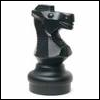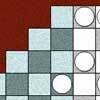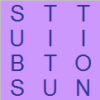Search by Topic

Resources tagged with Factors and multiples similar to Readme:

Filter by: Content type:
Age range:
Challenge level:

There are 44 results

Broad Topics > Numbers and the Number System > Factors and multiplesThe Public Key

Age 16 to 18 Challenge Level:

Find 180 to the power 59 (mod 391) to crack the code. To find the secret number with a calculator we work with small numbers like 59 and 391 but very big numbers are used in the real world for this.Dirisibly Yours

Age 16 to 18 Challenge Level:

Find and explain a short and neat proof that 5^(2n+1) + 11^(2n+1) + 17^(2n+1) is divisible by 33 for every non negative integer n.Powerful Factors

Age 16 to 18 Challenge Level:

Use the fact that: x²-y² = (x-y)(x+y) and x³+y³ = (x+y) (x²-xy+y²) to find the highest power of 2 and the highest power of 3 which divide 5^{36}-1.Factoring a Million

Age 14 to 16 Challenge Level:

In how many ways can the number 1 000 000 be expressed as the product of three positive integers?Expenses

Age 14 to 16 Challenge Level:

What is the largest number which, when divided into 1905, 2587, 3951, 7020 and 8725 in turn, leaves the same remainder each time?Big Powers

Age 11 to 16 Challenge Level:

Three people chose this as a favourite problem. It is the sort of problem that needs thinking time - but once the connection is made it gives access to many similar ideas.Divisibility Tests

Age 11 to 16Squaresearch

Age 14 to 16 Challenge Level:

Consider numbers of the form un = 1! + 2! + 3! +...+n!. How many such numbers are perfect squares?Data Chunks

Age 14 to 16 Challenge Level:

Data is sent in chunks of two different sizes - a yellow chunk has 5 characters and a blue chunk has 9 characters. A data slot of size 31 cannot be exactly filled with a combination of yellow and. . . .What a Joke

Age 14 to 16 Challenge Level:

Each letter represents a different positive digit AHHAAH / JOKE = HA What are the values of each of the letters?Phew I'm Factored

Age 14 to 16 Challenge Level:

Explore the factors of the numbers which are written as 10101 in different number bases. Prove that the numbers 10201, 11011 and 10101 are composite in any base.Different by One

Age 14 to 16 Challenge Level:

Make a line of green and a line of yellow rods so that the lines differ in length by one (a white rod)Times Right

Age 11 to 16 Challenge Level:

Using the digits 1, 2, 3, 4, 5, 6, 7 and 8, mulitply a two two digit numbers are multiplied to give a four digit number, so that the expression is correct. How many different solutions can you find?Star Product Sudoku

Age 11 to 16 Challenge Level:

The puzzle can be solved by finding the values of the unknown digits (all indicated by asterisks) in the squares of the $9\times9$ grid.Factorial

Age 14 to 16 Challenge Level:

How many zeros are there at the end of the number which is the product of first hundred positive integers?Multiplication Magic

Age 14 to 16 Challenge Level:

Given any 3 digit number you can use the given digits and name another number which is divisible by 37 (e.g. given 628 you say 628371 is divisible by 37 because you know that 6+3 = 2+7 = 8+1 = 9). . . .Transposition Cipher

Age 11 to 16 Challenge Level:

Can you work out what size grid you need to read our secret message?Public Key Cryptography

Age 16 to 18

An introduction to the ideas of public key cryptography using small numbers to explain the process. In practice the numbers used are too large to factorise in a reasonable time.Robotic Rotations

Age 11 to 16 Challenge Level:

How did the the rotation robot make these patterns?Really Mr. Bond

Age 14 to 16 Challenge Level:

115^2 = (110 x 120) + 25, that is 13225 895^2 = (890 x 900) + 25, that is 801025 Can you explain what is happening and generalise?Old Nuts

Age 16 to 18 Challenge Level:

In turn 4 people throw away three nuts from a pile and hide a quarter of the remainder finally leaving a multiple of 4 nuts. How many nuts were at the start?LCM Sudoku

Age 14 to 16 Challenge Level:

Here is a Sudoku with a difference! Use information about lowest common multiples to help you solve it.Take Three from Five

Age 14 to 16 Challenge Level:

Caroline and James pick sets of five numbers. Charlie chooses three of them that add together to make a multiple of three. Can they stop him?LCM Sudoku II

Age 11 to 18 Challenge Level:

You are given the Lowest Common Multiples of sets of digits. Find the digits and then solve the Sudoku.A Biggy

Age 14 to 16 Challenge Level:

Find the smallest positive integer N such that N/2 is a perfect cube, N/3 is a perfect fifth power and N/5 is a perfect seventh power.Modular Knights

Age 16 to 18 Challenge Level:

Try to move the knight to visit each square once and return to the starting point on this unusual chessboard.Sums of Squares and Sums of Cubes

Age 16 to 18

An account of methods for finding whether or not a number can be written as the sum of two or more squares or as the sum of two or more cubes.Factors and Multiples - Secondary Resources

Age 11 to 16 Challenge Level:

A collection of resources to support work on Factors and Multiples at Secondary level.Mod 3

Age 14 to 16 Challenge Level:

Prove that if a^2+b^2 is a multiple of 3 then both a and b are multiples of 3.Polite Numbers

Age 16 to 18 Challenge Level:

A polite number can be written as the sum of two or more consecutive positive integers. Find the consecutive sums giving the polite numbers 544 and 424. What characterizes impolite numbers?For What?

Age 14 to 16 Challenge Level:

Prove that if the integer n is divisible by 4 then it can be written as the difference of two squares.Diagonal Product Sudoku

Age 11 to 16 Challenge Level:

Given the products of diagonally opposite cells - can you complete this Sudoku?Sixational

Age 14 to 18 Challenge Level:

The nth term of a sequence is given by the formula n^3 + 11n . Find the first four terms of the sequence given by this formula and the first term of the sequence which is bigger than one million. . . .Napier's Location Arithmetic

Age 14 to 16 Challenge Level:

Have you seen this way of doing multiplication ?Number Rules - OK

Age 14 to 16 Challenge Level:

Can you convince me of each of the following: If a square number is multiplied by a square number the product is ALWAYS a square number...N000ughty Thoughts

Age 14 to 16 Challenge Level:

How many noughts are at the end of these giant numbers?Factorial Fun

Age 16 to 18 Challenge Level:

How many divisors does factorial n (n!) have?There's Always One Isn't There

Age 14 to 16 Challenge Level:

Take any pair of numbers, say 9 and 14. Take the larger number, fourteen, and count up in 14s. Then divide each of those values by the 9, and look at the remainders.Age 11 to 16 Challenge Level:

The items in the shopping basket add and multiply to give the same amount. What could their prices be?Telescoping Functions

Age 16 to 18

Take a complicated fraction with the product of five quartics top and bottom and reduce this to a whole number. This is a numerical example involving some clever algebra.Common Divisor

Age 14 to 16 Challenge Level:

Find the largest integer which divides every member of the following sequence: 1^5-1, 2^5-2, 3^5-3, ... n^5-n.Substitution Transposed

Age 11 to 16 Challenge Level:

Substitution and Transposition all in one! How fiendish can these codes get?Charlie's Delightful Machine

Age 11 to 16 Challenge Level:

Here is a machine with four coloured lights. Can you develop a strategy to work out the rules controlling each light?Factors and Multiples Game

Age 7 to 16 Challenge Level:

A game in which players take it in turns to choose a number. Can you block your opponent?